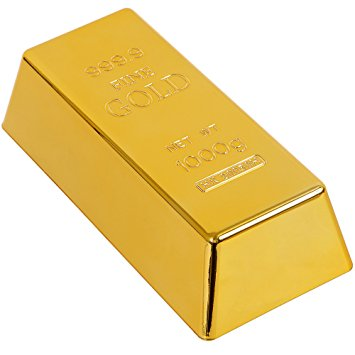Mahesh Godavarti
1Why is the decimal representation of 1/3 = 0.3333333... a never ending sequence of repeating 3s. Let's say you have a gold bar that you want to divide equally into three parts for yourself and your two friends. So, you need a cutting machine. However, the cutting machine can only cut whatever you feed it into 10 equal parts. So, what do you do? STEP 1: You feed the gold bar into the machine and it spits out 10 little bars each of the size equal to \frac{1}{10} gold bar. Each of you take 3 \frac{1}{10} gold bars. 1 is left. STEP 2: You feed the remaining \frac{1}{10} gold bar into the machine and get 10 smaller bars each of the size equal to \frac{1}{10} \cdot \frac{1}{10} gold bar = \frac{1}{100} gold bar. Each of you take 3 \frac{1}{100} gold bars. 1 is left. These 3 \frac{1}{100} gold bars go with the 3 \frac{1}{10} gold bars that you got from STEP 1. So, now you have 3 \frac{1}{10} gold bars + 3 \frac{1}{100} gold bars. STEP 3: You feed the remaining \frac{1}{100} gold bar into the machine and get 10 even smaller bars each of the size equal to \frac{1}{10} \cdot \frac{1}{100} gold bar = \frac{1}{1000} gold bar. Each of you take 3 \frac{1}{1000} gold bars. 1 is left. These 3 \frac{1}{100} gold bars go with 3 \frac{1}{10} gold bars + 3 \frac{1}{100} gold bars you got from STEP 2. So, now you have 3 \frac{1}{10} gold bars + 3 \frac{1}{100} gold bars + 3 \frac{1}{1000} gold bars. You get the idea. That you will never be able to get an exact 1/3 of the gold bar using a machine that can only give you ten equal parts. Decimal system is exactly that. You might wonder then if you had a machine that can divide the gold bar into three equal parts then you would be done in a single step. That is correct!